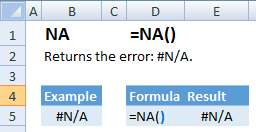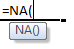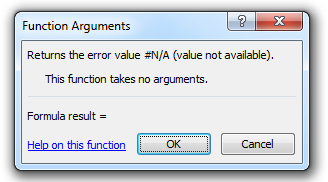### NA Function Examples – Excel & Google Sheets

This Excel Tutorial demonstrates how to use the Excel NA Function in Excel to create an #N/A error, with formula examples.

## NA Function Description:

The NA Function Returns the error: #N/A.

## Formula Examples:Example Formula Result
#N/A =NA() #N/A
AutoMacro - VBA Code Generator

## Syntax and Arguments:

The Syntax for the NA Formula is:

`=NA()`

## Additional Notes

Return to the List of all Functions in Excel

## How to use the NA Function in Excel:

To use the AND Excel Worksheet Function, type the following into a cell:
`=AND(`
After entering it in the cell, notice how the AND formula inputs appear below the cell:You will need to enter these inputs into the function. The function inputs are covered in more detail in the next section. However, if you ever need more help with the function, after typing “=NA(” into a cell, without leaving the cell, use the shortcut CTRL + A (A for Arguments) to open the “Insert Function Dialog Box” for detailed instructions:For more information about the NA Formula visit the
Microsoft Website.

## Excel Practice Worksheet

Practice Excel functions and formulas with our 100% free practice worksheets!

• Automatically Graded Exercises
• Learn Excel, Inside Excel!